# Will I ever be a bayesian statistician ? (part 1)

Last week, during the workshop on Statistical Methods for Meteorology and Climate Change (here), I discovered how powerful bayesian techniques could be, and that there were more and more bayesian statisticians. So, if I was to fully understand applied statisticians in conferences and workshops, I really have to understand basics of bayesian statistics. I have published some time ago some posts on bayesian statistics applied to actuarial problems (here or there), but so far, I always thought that bayesian was a synonym for magician. To be honest, I am a Muggle, and I have not been trained as a bayesian. But I can be an opportunist…

So I decided to publish some posts on bayesian techniques, in order to prove that it is actually not that difficult to implement.As far as I understand it, in bayesian statistics, the parameter is considered as a random variable (which is also the case, in classical mathematical statistics). But here, here assume that this parameter does have a parametric distribution….
Consider a classical statistical problem: assume we have a sample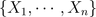i.i.d. with distribution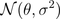. Here we note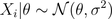since parameteris a random variable. The idea is to assume thathas a (so called a priori) distribution, e.g.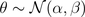So far it was simple. The idea is then to consider the posterior distribution of, given the observations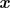. Thus, we need to compute the distribution of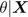which is here extremely simple (due to properties of the Gaussian distribution), i.e.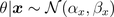where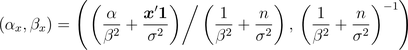And them, it becomes extremely natural to consider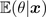as an estimator of given our sample data (and thus, we also have a confidence interval since we know the distribution ofgiven the observations).
In order to be sure that we understood, consider now a heads and tails problem, i.e.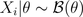. Note, first, that \theta has support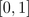. So we need a distribution on that support. Why not a beta distribution ? E.g.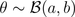Thus,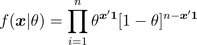and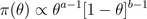From Bayes formula,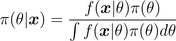and we get easily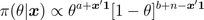which is the density of a Beta distribution, i.e.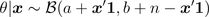prior=dbeta(u,a,b)
posterior=dbeta(u,a+y,n-y+b)

The estimator proposed is then the expected value of that conditional distribution,Note that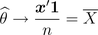Further, it is possible to derive confidence intervals using quantiles of the posterior distribution.
On the graphs below, we consider the following heads/tails sampleA first idea is to consider a uniform prior distribution.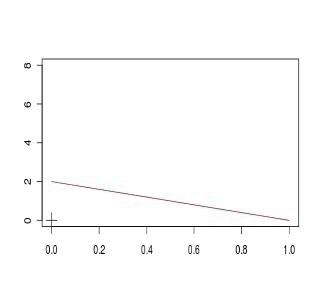A second idea is to consider an asymmetric beta distribution. First, with an asymmetry on the left,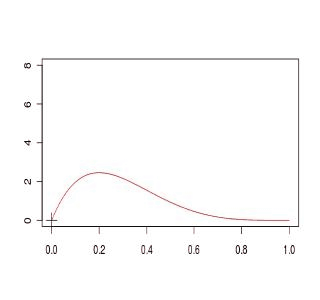or on the right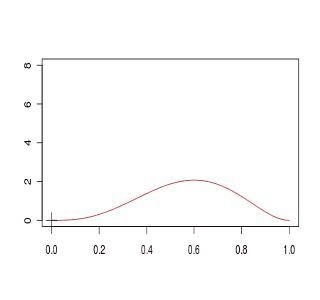Finally a third idea is simply to get back to the standard Gaussian approximation,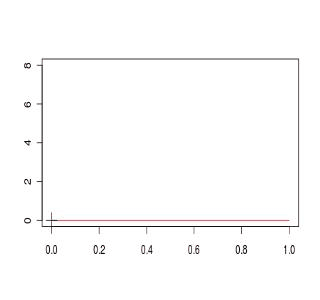If we compare the four models, we obtain (the plain black line is the Gaussian approximated distribution for the empirical mean), and red lines are obtained from prior beta distributionsThe code to generate those graphs is the following
a1=1; b1=1
D1[1,]=dbeta(u,a,b)
a2=4; b2=2
D2[1,]=dbeta(u,a,b)
a3=2; b3=4
D3[1,]=dbeta(u,a,b)
setseed(1)
S=sample(0:1,size=100,replace=TRUE)
COULEUR=rev(rainbow(120))
D1=D2=D3=D4=matrix(NA,101,length(u))
for(s in 1:100){
y=sum(S[1:s])
D1[s+1,]=dbeta(u,a1+y,s-y+b1)
D2[s+1,]=dbeta(u,a2+y,s-y+b2)
D3[s+1,]=dbeta(u,a3+y,s-y+b3)
D4[s+1,]=dnorm(u,y/s,sqrt(y/s*(1-y/s)/s))
plot(u,D1[1,],col="black",type="l",ylim=c(0,8),
xlab="",ylab="")
for(i in 1:s){lines(u,D1[1+i,],col=COULEUR[i])}
points(y/s,0,pch=3,cex=2)
plot(u,D2[1,],col="black",type="l",ylim=c(0,8),
xlab="",ylab="")
for(i in 1:s){lines(u,D2[1+i,],col=COULEUR[i])}
points(y/s,0,pch=3,cex=2)
plot(u,D3[1,],col="black",type="l",ylim=c(0,8),
xlab="",ylab="")
for(i in 1:s){lines(u,D3[1+i,],col=COULEUR[i])}
points(y/s,0,pch=3,cex=2)
plot(u,D4[1,],col="white",type="l",ylim=c(0,8),
xlab="",ylab="")
for(i in 1:s){lines(u,D4[1+i,],col=COULEUR[i])}
points(y/s,0,pch=3,cex=2)
plot(u,D4[s+1,],col="black",lwd=2,type="l",
ylim=c(0,8),xlab="",ylab="")
lines(u,D1[1+i,],col="blue")
lines(u,D2[1+i,],col="red")
lines(u,D3[1+i,],col="purple")
points(y/s,0,pch=3,cex=2)
}

Here, we can see that computations are simple if the prior distribution has a distribution which is the conjugate of the observations’ distribution (see here for the list of prior and posterior standard distributions).
So far, I have two questions that naturally show up

• is it possible to start with a neutral prior distribution, non informative ?
• what if we are no longer working with conjugate distributions ?

Well, I guess I have to work a bit more to answer those questions…. to be continued# Convergences de variables aléatoiresLe schéma – ou diagramme – des relations entre les modes convergences ressemble à ce qui est présenté sur la droite. C’est grosso modo ce que j’ai dit en cours (sans le représenter explicitement).

Je ne vais pas démontrer ces implications car ce sont des théorèmes (qui peuvent donc se trouver dans n’importe quel textbook de probabilité).

En revanche, un étudiant m’avait demandé si les réciproques étaient vraies. Comme toujours en maths, si les réciproques étaient toujours vraies, on le mettrait. Donc ici les réciproques sont généralement fausses. On peut toutefois se demander quand ces réciproques peuvent être vraies…et quand elles sont fausses.

• de la convergence en proba à la convergence en moyenne

Commençons par le plus simple (à mon avis). Ilexiste un cas particulier où cette réciproque est vraie: le cas de variables aléatoires bornées. Autrement dit, si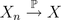et que l’on a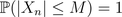pour tout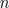, alors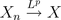pour tout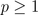.
Bon, en fait la condition est un peu trop forte, et il suffit que la suite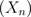soit uniformément intégrable.
Maintenant, il existe aussi des cas où cette réciproque sera fausse (et on se doute qu’en construisant une suite non bornée, on devrait y arriver). Par exemple, supposons que pour tout,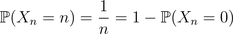On peut montrer que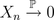, car en effet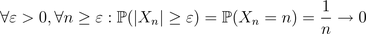Toutefois, certes la suite converge dans L1, mais vers une limite différente, étant donné que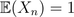pour tout. De plus,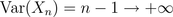et donc la suite ne converge pas dans L2.

• de la convergence en loi à la convergence en probaUn exemple simple ici: siconverge en loi vers une constante, alors on aura convergence en probabilité (vers la même constante).
Maintenant la réciproque est relativement simple, l’idée étant quen’implique en rien, au sens où, pour tout. Supposons maintenant que l’on ait à la foiset, où on va supposer que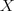est une loi symétrique. La symétrie signifie que. Notons que l’on a alors forcément. Supposons que la réciproque soit toujours vraie, alors on devrait avoir, et donc on aurait que, ce qui n’arrive que siest la constante.

• de la convergence en proba vers la convergence presque sûre

Je ne vais pas m’étendre sur la construction d’exemples pour lesquels la réciproque est fausse (formellement, il suffit de changer d’espace de probabilité sous-jacent, je peux renvoyer vers les notes de cours de Philippe Briand (ici) et Hélène m’a suggéré également les exercices en ligne ). Par contre, il existe des cas importants où la réciproque est vraie.
Considérons une suite de variables indépendantes et identiquement distribuées. Posons alors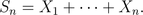On peut alors montrer que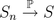si et seulement si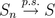(ça doit être du Borel-Cantelli).

• de la convergence en loi à la convergence presque sûre

Je ne vais pas non plus m’étendre davantage, mais on a un résultat qui ressemble à ce que je disais auparavant. En fait, il existe un théorème (dit de Skorohod) qui permet de dire que si on a une convergence en loi, alors on peut construite une suite de variables aléatoires qui
convergera presque sûrement. Formellement on a que si, alors il existe une suite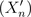et une variable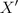, telles que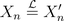et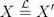telles que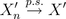. Mais de manière générale, si la suite n’est pas construite comme il faut (paren quelques sortes) alors il est assez rare d’avoir la réciproque.

# Un peu de terminologie statistiqueSuite à quelques questions (anodines) posées au fil du cours de statistique, je prends 5 minutes pour refaire le point sur les abréviations qui me semblent classiques, mais qui le ne sont peut-être pas (car je n’ai pas l’habitude d’enseigner en deuxième année, pour ceux qui en doutaient encore).

Tout d’abord des locutions latines. Par exemple i.e. n’est pas Internet Explorer mais vient du latin id est et signifie donc c’est-à-dire. Parmi les petites locutions proches, e.g. vient du latin exempli gratia, et signifie littéralement par la grâce de l’exemple mais on retiendra que cela signifie par exemple.

A l’occasion, il m’arrive d’utiliser ca qui est une abréviation du latin circa, signifiant environ.Et quid signifie en latin quoi. Sinon dans les textbook, q.e.d. vient du latin quod erat demonstrandum, que l’on traduit souvent par ce qu’il fallait démontrer, ou cqfd. Enfin, vs est une contraction du latin versus signifiant comparé à. Bon, je pense avoir fait le tour pour les expressions latines.

Du côté des lettres grecques couramment utilisées en statistique, α i.e. alpha, et β i.e. beta, que l’on verra apparaître dans les erreurs de tests statistiques. Γ est une fonction qu’il pourrait être utile d’utiliser dans les calculs d’intégrales (correspondant à une généralisation de la factorielle). δ i.e. delta, apparaît dans la notation de la masse de Dirac. ε i.e. epsilon que l’on retrouve un peu partout en analyse pour dénoter une constante qui peut être aussi petite qu’on le souhaite. θ i.e. theta qui correspond au paramètre de nos lois, mais parfois on utilisera aussi λ i.e. lambda qui apparaît comme paramètre de la loi de Poisson ou de la loi exponentielle. μ i.e. mu qui désigne généralement la moyenne d’une variable aléatoire, ν i.e. nu qui désigne les degrés de liberté dans les lois de Student ou de Fisher. ρ i.e. rho qui désigne parfois la corrélation. σ i.e. sigma qui désigne l’écart-type d’une variable aléatoire. χ i.e. khi (ou chi) qui apparaît souvent avec une puissance 2, dans la loi du chi-deux. Et enfin ω i.e. omega qui désigne un état de la nature, appartenant à Ω (la lettre majuscule).

Voilà pour le premier point de terminologie. Je me laisse la possibilité de poursuivre, en particulier sur les abréviations plus mathématiques (comme les p.s. ou en anglais a.s., ou autres w.r.t.). Toutes les suggestions sont les bienvenues…